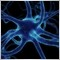How to change chart period on code base in oncalculate function for custom indicator?277

Hello,

By trying to switch chart period within oncalculate function in a custom indicator, we use following code below. But the chart automatically closes after the return. How can this be solved? Thank you.

ENUM_TIMEFRAMES periods[] = {PERIOD_M30, PERIOD_H1};
int period_ind;

int OnCalculate(const int rates_total,const int prev_calculated,
const datetime &Time[],
const double   &Open[],
const double   &High[],
const double   &Low[],
const double   &Close[],
const long     &TickVolume[],
const long     &Volume[],
{
...
...
...
long currChart=ChartFirst();
ENUM_TIMEFRAMES period = periods[period_ind % (ArraySize(periods))];
ChartSetSymbolPeriod(currChart, ChartSymbol(currChart), period) ;

return(rates_total);
}1025

zeno:

Hello,

By trying to switch chart period within oncalculate function in a custom indicator, we use following code below. But the chart automatically closes after the return. How can this be solved? Thank you.

Programmatically change the current chart timeframe impossible.

But you can create a new chart using ChartOpen .39017

zeno:

Hello,

By trying to switch chart period within oncalculate function in a custom indicator, we use following code below. But the chart automatically closes after the return. How can this be solved? Thank you.

Do you really want to change the chart period on each tick ?39017

Fleder:

Programmatically change the current chart timeframe impossible.

But you can create a new chart using ChartOpen .

Yes it's possible to change the current chart timeframe.